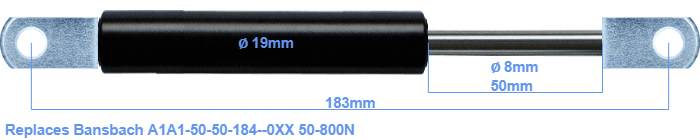# Replacement for Bansbach A1A1-50-50-184–0XX 50-800N

\$26.01

Replacement gas spring for the Bansbach A1A1-50-50-184--0XX 50-800 Newton. The eye has a thickness of 10mm and a hole diameter of 8.1mm. Brand: Stabilus Industry Line.
 Force Choose an option50 Newton60 Newton80 Newton100 Newton120 Newton140 Newton150 Newton160 Newton180 Newton200 Newton220 Newton240 Newton250 Newton260 Newton280 Newton300 Newton320 Newton340 Newton350 Newton360 Newton380 Newton400 Newton420 Newton440 Newton450 Newton460 Newton480 Newton500 Newton520 Newton540 Newton550 Newton560 Newton580 Newton600 Newton620 Newton640 Newton650 Newton660 Newton680 Newton700 Newton720 Newton740 Newton750 Newton760 Newton780 Newton800 NewtonClearThis gas spring has a cylinder diameter of 19 mm. The rod has a diameter of 8 mm. The chrome part is called the rod. It has a length of 50 mm. The total length of this replacement is exactly 183 mm. This is the total distance between the centers of the two mounting parts. The distance from thread to thread (without mounting parts) is 145 mm. This replacement gas spring (A1A1-50-50-184--0XX) has a force of 50-800 Newton (N). This gas spring can replace a Bansbach gas spring, but is not an orignal Bansbach one. This gas spring was produced by Stabilus subsidiary Hahn with the brand Stabilus Industry Line. Nevertheless, this gas spring can be used as replacement gas spring, because the dimensions and forces are equal.